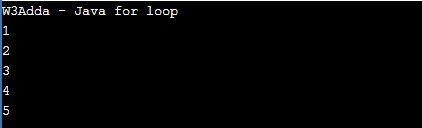# Java for loop

In this tutorial you will learn about the Java for loop and its application with practical example.

## Java for Loop

The for loop is used when we want to execute a block of code known times. In Java, the basic for loop is similar to what it is in C and C++. The for loop takes a variable as an iterator and assigns it with an initial value, and iterates through the loop body as long as the test condition is true. Once the loop statements are executed for the current iteration, the iterator is updated with a new value and if the test condition is still valid, we loop another time. When the test condition returns a false value, the for loop is ended.

Syntax:-

Above is the general syntax of a for a loop. We just initialize the iterator, check the condition and then increment or decrement the iterator value. The for loop has three components separated by semicolons.

initialization:- In this section is used to declare and initialize the loop iterator. This is the first statement to be executed and executed only once at the beginning.

condition:- Next, the test condition is evaluated before every iteration, and if it returns a true value then the loop body is executed for the current iteration. If it returns a false value then the loop is terminated and the control jumps to the next statement just after the for a loop.

incr/decr:- Once, the loop body is executed for the current iteration then the incr/decr statement is executed to update the iterator value and if the test condition is evaluated again. If it returns a true value then the loop body is executed another time. If the test condition returns a false value then the loop is terminated and the control jumps to the next statement just after the for a loop.

## Java For Loop Flow DiagramExample:-

Here, we have initialized ctr with an initial value of 1, in the next, we check the condition ctr <= 5 for each of the iterations. If the test condition returns True, the statements inside the loop body are executed and if the test condition returns False then the for loop is terminated. In the incr/decr section we have incremented the ctr value by one for each iteration. When we run the above c++ program, we see the following output –

Output:-## Java Infinite For Loop

When a loop executes repeatedly and never stops, it is said to be infinite for loop. In Java, if we leave the “initialization”, “increment” and “condition” statement blank, then the for loop will become an infinite loop.

Syntax:-

Example:-

In this tutorial we have learn about the Java for loop and its application with practical example. I hope you will like this tutorial.